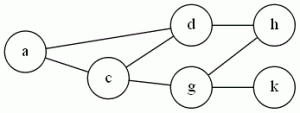# Clique

G&J don’t even bother to prove Clique is NP-Complete, just stating that it (and Independent Set) is a “different version” of Vertex Cover.  But the problem comes up often enough that it’s worth seeing in its own right.

The problem: Clique (I’ve also seen “Max Clique” or “Clique Decision Problem” (CDP))

The definition: Given a graph G=(V,E), and a positive integer J (G&J say J needs to be ≤ |V|, but I think you can allow large values and make your algorithm just say “no”).  Does J contain a subset V’ of V, or size at least J, where every two vertices in V’ are joined by an edge in E?

Example:  Using the graph we had for VC:{a,c,d} form a clique of size 3.  There are no cliques of size 4, but if we add the edge (d,g) and the edge (c,h), then the vertices {c,d,g,h} would be one.

The reduction: From Vertex Cover.  Given a graph G and an integer K that is an instance of Vertex Cover, take the complement of G (the complement of a graph has the same vertices, but the set of edges is “inverted”:  (u,v) is an edge in the complement if and only if it was not an edge in G), and use it as your instance of CDP.  Set J= |V|-K

Difficulty: 3.  It’s not a 2 because it takes a little thought to see why the complement works.

Note: As stated perviously, I teach this course using the Cormen book, so in class I show Clique is NP-Hard by reducing from 3SAT.  It’s a good example of a hard reduction, probably about as hard as the VC reduction G&J do.

### 2 responses to “Clique”

1.Pradeep Raju

There are no cliques of size 4, but if we add the edge (d,g), then the vertices {c,d,g,h} would be one.

It would be right if we have two edges (d,g) and (c,h).

2.Sean McCulloch

oops, I’ll fix it now. Thanks!